# Trigonometry

Theorem of Pythagoras

The theorem of Pythagoras says that the largest side squared is equal to the sum of the other two sides squared. The largest side is called the hypotenuse and is always opposite the 90° angle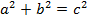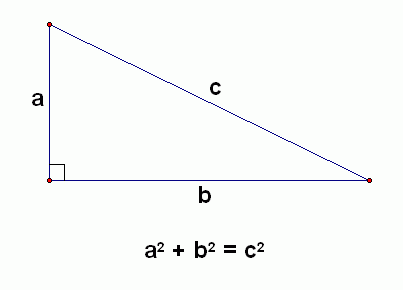In right-angle triangles special ratios sin, cos and tan exist between the angles and the lengths of the sides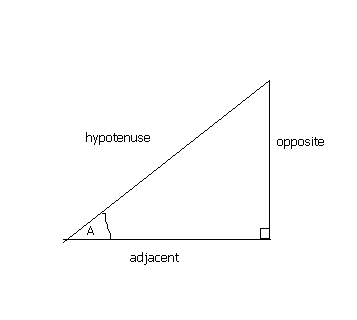Sin A =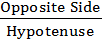Cos A =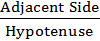TanA =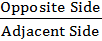If we’re trying to find the angle A we use the functions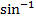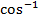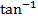A = ( )A = ( )A = ( )Minutes are the same as degrees except the...